# 机器学习【十一】管道模型

• 管道模型的基本概念和使用
• 使用管道模型进行模型选择
• 使用管道模型进行参数调优

1.基本概念

#导入数据集生成器
from sklearn.datasets import make_blobs
#导入数据集拆分工具
from sklearn.model_selection import train_test_split
#导入预处理工具
from sklearn.preprocessing import StandardScaler
#导入多层感知器神经网络
from sklearn.neural_network import MLPClassifier
#导入画图工具
import matplotlib.pyplot as plt
#生成样本数量200，分类2，标准差5的数据集
X,y = make_blobs(n_samples=200,centers=2,cluster_std=5)
X_train,X_test,y_train,y_test = train_test_split(X,y,random_state=38)
#对数据进行预处理
scaler = StandardScaler().fit(X_train)
X_train_scaled = scaler.transform(X_train)
X_test_scaled = scaler.transform(X_test)
print('训练集数据形态：',X_train_scaled.shape)
print('测试集数据形态：',X_test_scaled.shape)
训练集数据形态： (150, 2)

#这段代码中，我们选择的是MLP多层感知神经网络作为下一步要用的分类器模型【因为MLP是典型的需要进行数据预处理的算法模型】

【结果分析】

训练集中的数据样本为150个，测试集中的样本数量为50个，特征数都是2

#原始的训练集
plt.scatter(X_train[:,0],X_train[:,1])
#经过预处理的训练集
plt.scatter(X_train_scaled[:,0],X_train_scaled[:,1],marker='^',edgecolor='k')
plt.title('training set & scaled trianing set')
plt.show()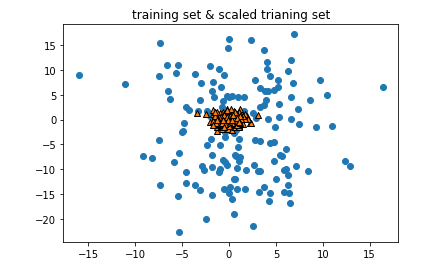【结果分析】

从图中可以看到，StandardScaler将训练集的数据变的更加聚拢——> 利于使用神经网络模型进行拟合

【本例中选择hidden_layer_sizes 和 alpha 进行实验】

#导入网格搜索
from sklearn.model_selection import GridSearchCV
#设定网格搜索的模型参数字典
params = {'hidden_layer_sizes':[(50,),(100,),(100,100)],'alpha':[0.0001,0.001,0.01,0.1]}
#建立网格搜索模型
grid = GridSearchCV(MLPClassifier(max_iter=1600,random_state=38),param_grid=params,cv=3)
#拟合数据
grid.fit(X_train_scaled,y_train)
print('最佳得分：',grid.best_score_)
print('最佳参数',grid.best_params_)
最佳得分： 0.9533333333333334

print('测试集得分：',grid.score(X_test_scaled,y_test))
测试集得分： 0.82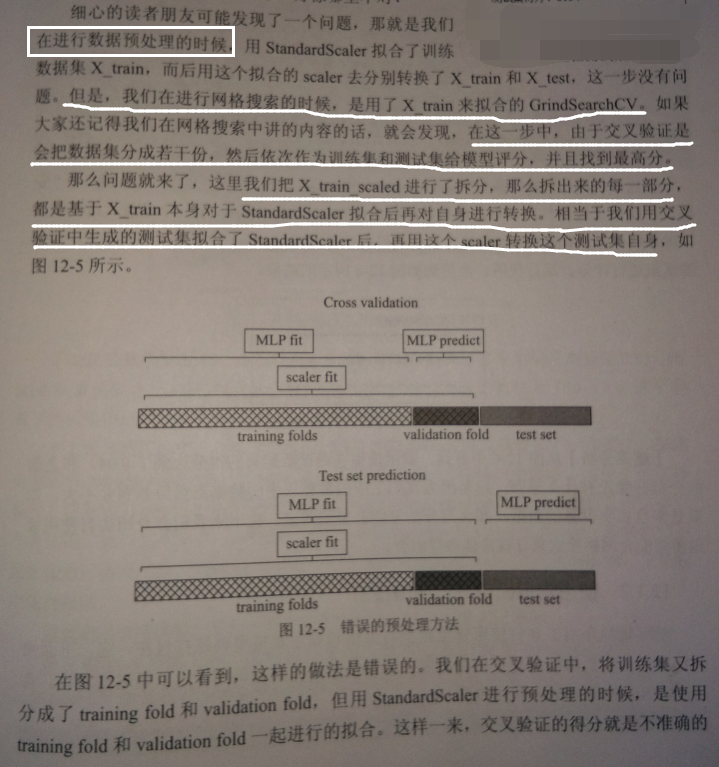由于想测试的参数组合有3*4=12个，需要做12次预处理吗？

#导入管道模型
from sklearn.pipeline import Pipeline
#建立包含预处理和神经网络的管道模型
pipeline = Pipeline([('scaler',StandardScaler()),('mlp',MLPClassifier(max_iter=1600,random_state=38))])
#拟合数据
pipeline.fit(X_train,y_train)
print('使用管道模型的MLP模型评分：',pipeline.score(X_test,y_test))
使用管道模型的MLP模型评分： 0.82

【结果分析】

2.使用管道模型进行网格搜索

刚使用管道模型将 数据预处理和模型训练打包 到了一起

下面，进行网格搜索：

#设置参数字典
params = {'mlp__hidden_layer_sizes':[(50,),(100,),(100,100)],'mlp__alpha':[0.0001,0.001,0.01,0.1]}
#将管道模型加入网格搜索
grid = GridSearchCV(pipeline,param_grid=params,cv=3)
#拟合数据
grid.fit(X_train ,y_train)
print('交叉验证最高分',grid.best_score_)
print('最优参数',grid.best_params_)
print('测试集得分',grid.score(X_test,y_test))
交叉验证最高分 0.8533333333333334

【随机生成的数据集不同，上述值不同】

【结果分析】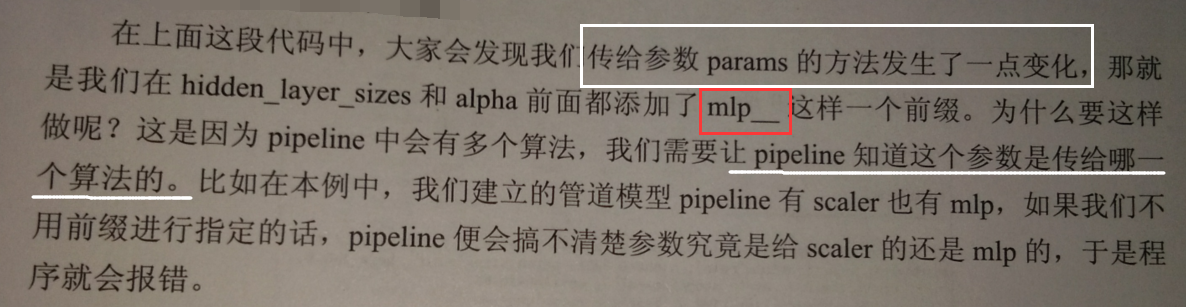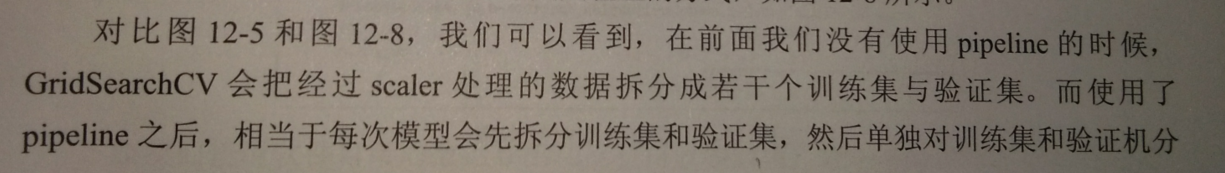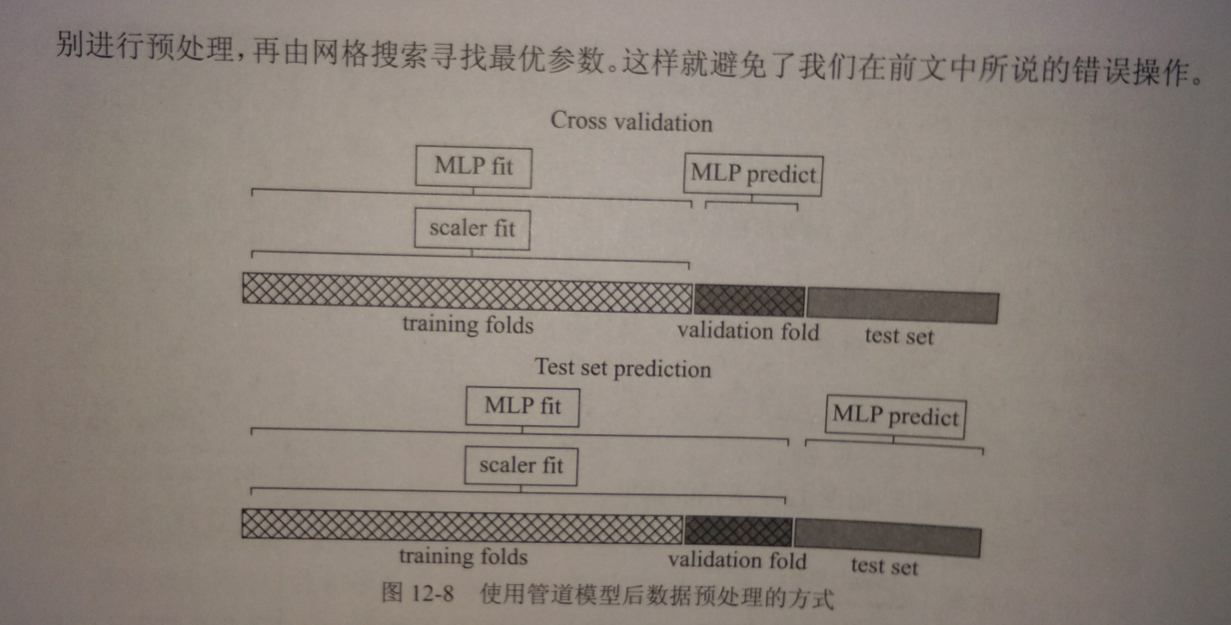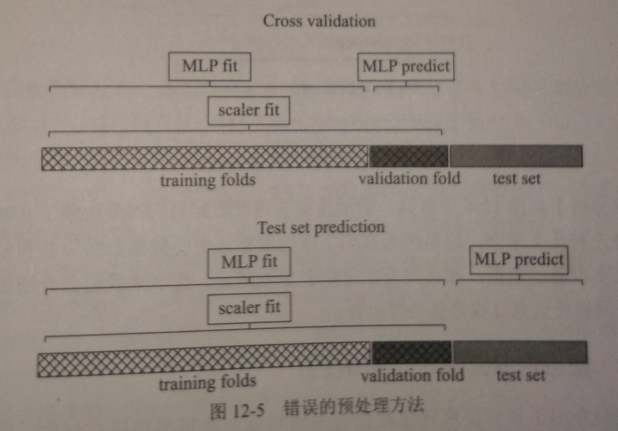print(pipeline.steps)
[('scaler', StandardScaler(copy=True, with_mean=True, with_std=True)), ('mlp', MLPClassifier(activation='relu', alpha=0.0001, batch_size='auto', beta_1=0.9,
beta_2=0.999, early_stopping=False, epsilon=1e-08,
hidden_layer_sizes=(100,), learning_rate='constant',
learning_rate_init=0.001, max_iter=1600, momentum=0.9,
n_iter_no_change=10, nesterovs_momentum=True, power_t=0.5,
validation_fraction=0.1, verbose=False, warm_start=False))]

【结果分析】

pipeline.steps 把包含在管道模型中的数据预处理scaler和多层感知神经网络MLP的全部参数返回，如同流水线的工作流程

posted @ 2019-05-08 12:11  远征i  阅读(718)  评论(0编辑  收藏